## Sub-Threshold Conduction of a Power MOSFET

The banner line description of the BUK9535 power MOSFET is given as,

Logic level N-channel enhancement mode Field-Effect Transistor (FET) in a plastic package using TrenchMOS technology

In the sub-threshold region we can fit the drain current to the following basic exponential model,

$$I_D =I_S \exp\left( \dfrac{V_{GS}}{\eta V_T}\right)$$

## Darlington Current Gain

The darlington transistor arrangement consists of 2 cascaded bipolar transistors, in such a manor that the forward current gain of the first transistor is multiplied by the second.  A schematic of a PNP darlington transistor arrangement is shown in the figure below.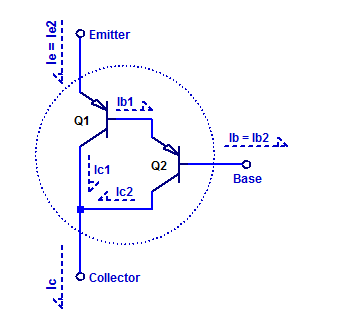## Linearity of a DAC0800 Current Output R2R DAC

The DAC0800 is a current output R-2R DAC with 8 bits of resolution. As part of a solar panel monitor, the DAC0800 serves as a programmable reference for a shunt regulator. The output of the DAC is mirror 1:1 into a $$5\;k\Omega$$ load; forming the voltage reference for the shunt regulator. A simplified schematic of the DAC is shown below.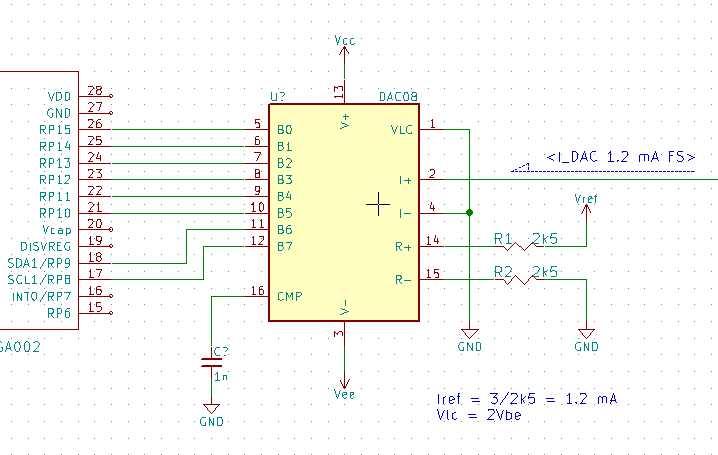## NiCd Fast-Charge Profile

In this post, the charge profile of a Panasonic N-700AACL NiCd battery will be instrumented.

The two recommended termination signatures to be used when fast-charging NiCd cells are either negative $$\Delta V$$ or positive dT/dt. Thermal detection is more challenging to implement as one requires good thermal contact between a temperature detector and the cell under charge. In the case of negative $$\Delta V$$ one already has the cell potential by the same contacts being used to apply the charge current. However, one requires sufficient measurement precision of the cell under charge to detect the relatively small  $$\Delta V$$ signature.

The charge profile of an N-700 cell during various charge rates is shown in the figure below.

## CD40106 Oscillator

In this post a sample schmitt-trigger relaxation oscillator is constructed and instrumented.

## Measurement Setup

A simplified schematic of the test setup is shown in the figure below.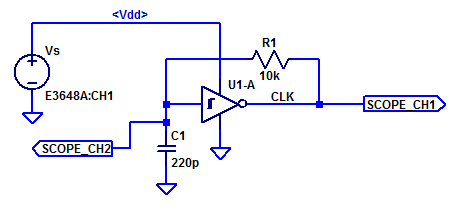Continue reading “CD40106 Oscillator”

## 1N4148 Diode Reverse Biased Junction Capacitance

Most of the datasheets from assorted suppliers of a 1N4148 diode all guarantee a maximum diode capacitance of 4 pF at $$V_D$$ = 0 VDC. A sample excerpt from the Vishay datasheet has the $$C_D$$ specification circled in red.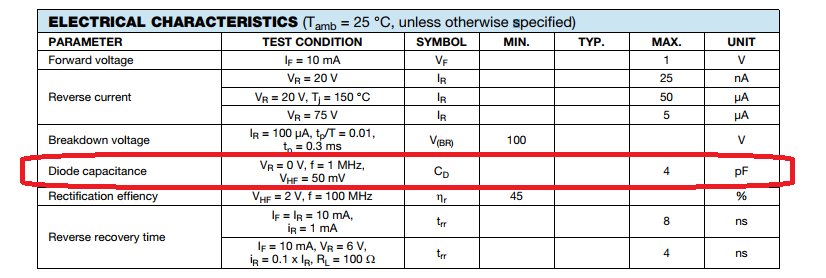Continue reading “1N4148 Diode Reverse Biased Junction Capacitance”

## CD40106 Output Drive Characteristics

In this post the output drive characteristics of a Cd40106 are measured and analyzed. The drive strength of a gate is important in high-speed signaling in order to quickly charge/discharge PCB interconnect capacitance and the effective input capacitance of the receiving gates/ICs.

The datasheet’s specification for the CD40106’s output low current sink $$I_{OL}$$ is shown in the figure below.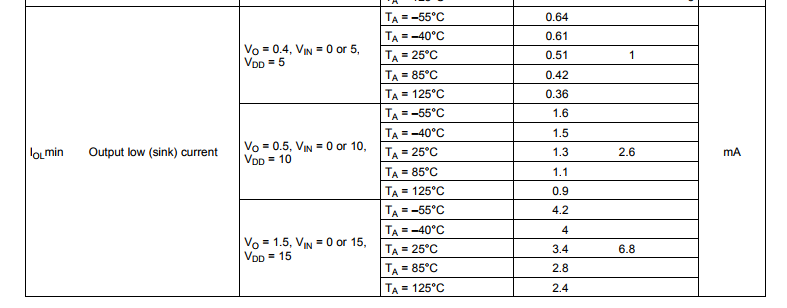We can translate the typically sink current to an approximate resistance as,

$$R_{OL,5} = \dfrac{V}{I} = {0.4}{0.001 } = 400 \; \Omega$$

From the datasheet we can calculated the typical output resistance for all 3 specified Vdd supply values as,

$$R_{OL,5} = 400 \; \Omega \;\;\; R_{OL,10} = 192 \; \Omega \;\;\;R_{OL,15} = 221 \; \Omega$$

Note the output resistance is specified to be lower on a 10 V supply versus a 15 V supply. In the section below we will determine if indeed a 10 V supply yields the lowest output low resistance. Continue reading “CD40106 Output Drive Characteristics”

## Schmitt-Trigger Threshold Levels (CD40106)

A schmitt-trigger to a first operates just like any digital buffer/inverter where a logic level input ( Gnd or Vdd ) yields some digital output value based on the logic function of the gate. What is different about an inverting schmitt-trigger over a basic inverter is the input threshold voltage changes depending on the gate’s output state. In the case of a schmitt-trigger the input threshold level receives a small positive feedback from the output. Hence when the output is triggered high, the input threshold voltage is boosted a small amount. Equivalently, when the output is low the input threshold voltage is lowered a small amount. This positive feedback of the output to the input is refereed to as hysteresis.

The transfers characteristics figure available from the  CD40106 datasheet is shown below.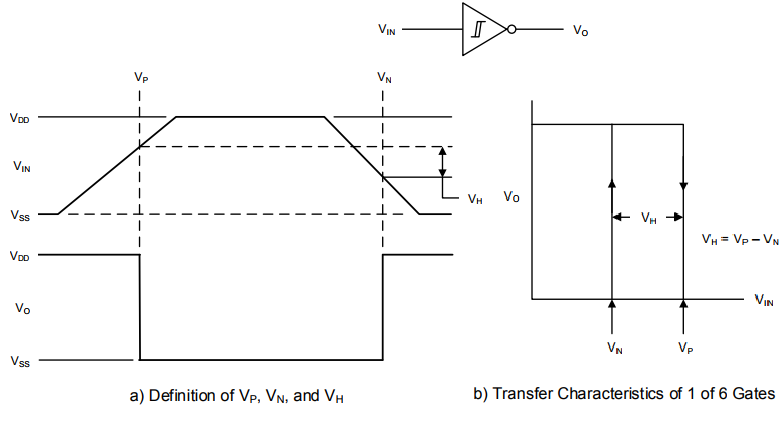## 1N4148 Diode Forward Biased I-V Curve

In this blog post, the forward biased voltage-current relationship of a 1N4148 diode will be measured and analyzed.

Shockley in 1949, published a paper describing the behavior of a diode as,

$$I_D = I_s \left( \exp \left( \dfrac{V_D}{\eta V_T}\right) -1 \right)$$

Where $$\eta$$ is a constant representing the ideality of device, typical values of $$\eta$$ range from 1 to 2 for silicon diodes.

$$V_T = \dfrac{kT}{q}$$

At a room temperature of $$22\;^o\text{C}$$ the thermal voltage is,

$$V_T(22\;^o\text{C}) = \dfrac{(1.381\cdot 10^{-23})(273.15+22)}{1.602\cdot 10^{-19}} = 25.4 \text{ mV}$$ Continue reading “1N4148 Diode Forward Biased I-V Curve”